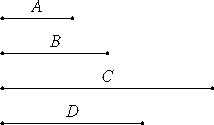# Proposition 4

If a cubic number multiplied by a cubic number makes some number, then the product is a cube.

Let the cubic number A multiplied by the cubic number B make C.

I say that C is cubic.IX.3

Multiply A by itself to make D. Then D is a cube.

Since A multiplied by itself makes D, and multiplied by B makes C, therefore A is to B as D is to C. And, since A and B are cubic numbers, therefore A and B are similar solid numbers. Therefore two mean proportional numbers fall between A and B, so that two mean proportional numbers fall between D and C also.

VIII.23

And D is a cube, therefore C is also a cube.

Therefore, if a cubic number multiplied by a cubic number makes some number, then the product is cubic.

Q.E.D.

## Guide

Of course, m3n3 = (mn)3.